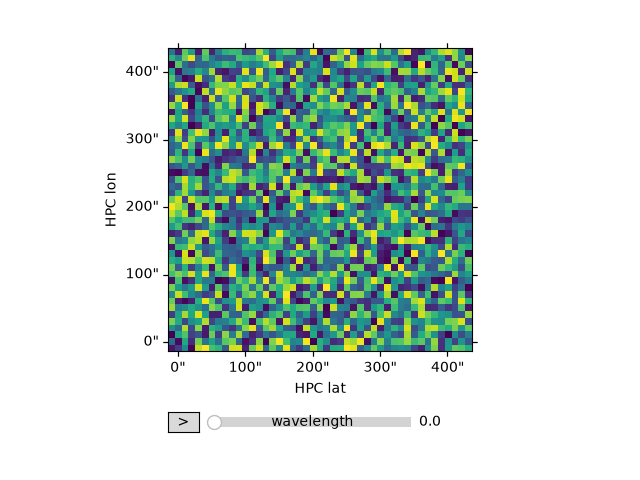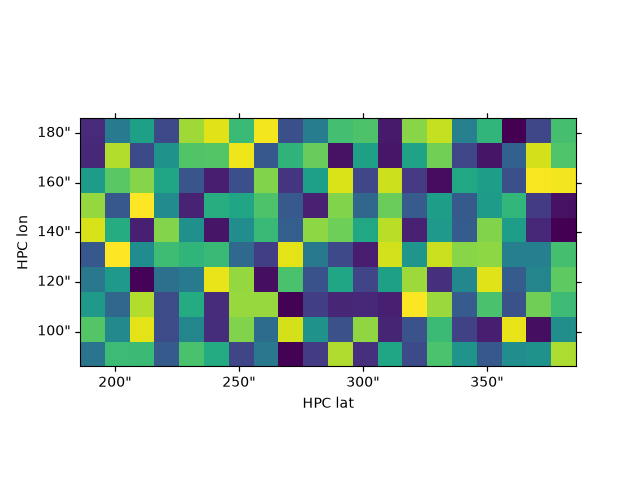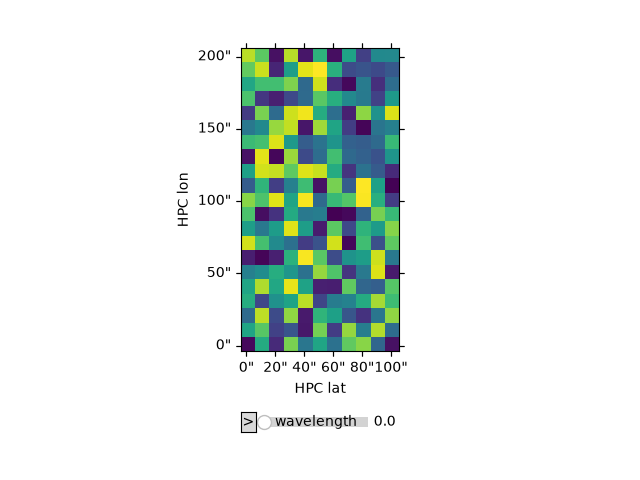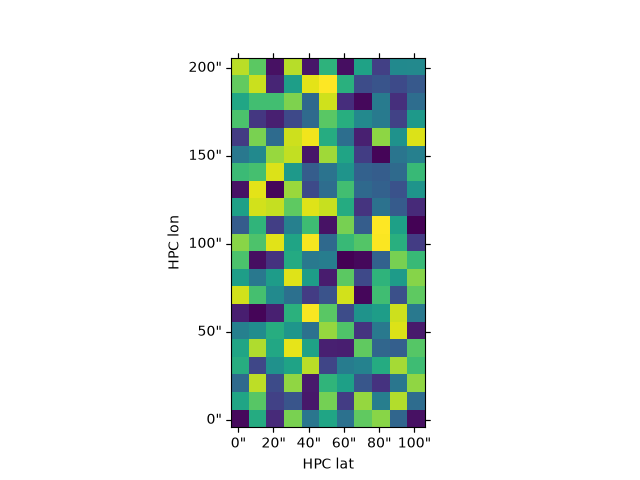# Examples of cropping an NDCube#

One of the powerful aspects of having coordinate-aware data stored as an `NDCube` is the ability to crop and slice the data and coordinates in a standardised and easy way.

For example, there may be a region of interest you would like to crop out along a certain dimension of your cube. In this example, this method to slice an `NDCube` are illustrated.

```import astropy.wcs
import numpy as np
from astropy import units as u
from astropy.coordinates import SkyCoord, SpectralCoord
from sunpy.coordinates import frames

from ndcube import NDCube
```

Let’s begin by creating an example `NDCube` object. For this case, we’ll generate an `NDCube` that consists of 3 dimensions space-space-wavelength. This is analogous to an observation including images in multiple wavelengths. Lets first define a 3-D numpy array, and then define the WCS information that describes the data. We’ll just create an array of random numbers, and a WCS which consists of the coordinate information which in this case will be in Helioprojective (i.e. an observation of the Sun) in latitude and longitude, and over several wavelengths in the range of 10.2 - 11 angstrom.

```# Define the data of random numbers. Here the spatial dimensions are (45, 45) and the wavelength dimension is 5.
data = np.random.rand(5, 45, 45)
# Define the WCS
wcs = astropy.wcs.WCS(naxis=3)
wcs.wcs.ctype = 'HPLT-TAN', 'HPLN-TAN', "WAVE"
wcs.wcs.cunit = 'arcsec', 'arcsec', 'Angstrom'
wcs.wcs.cdelt = 10, 10, 0.2
wcs.wcs.crpix = 2, 2, 0
wcs.wcs.crval = 1, 1, 10
wcs.wcs.cname = 'HPC lat', 'HPC lon', 'wavelength'
# Instantiate the `~ndcube.NDCube`
example_cube = NDCube(data, wcs=wcs)
```

So we now have created an `NDCube` named `example_cube`. You may have noticed that the order of the WCS is reversed to the array order - this is normal convention, and something to remember throughout. Now let’s first inspect the cube.

Here we can inspect the cube by plotting it

```example_cube.plot()
``````<mpl_animators.wcs.ArrayAnimatorWCS object at 0x7fa751ff6710>
```

We can also inspect the dimensions of the cube:

```example_cube.dimensions
```
```<Quantity [ 5., 45., 45.] pix>
```

We can also inspect the world coordinates for all array elements:

```example_cube.axis_world_coords()
```
```/home/docs/checkouts/readthedocs.org/user_builds/ndcube/conda/latest/lib/python3.11/site-packages/astropy/wcs/wcsapi/fitswcs.py:533: AstropyUserWarning: target cannot be converted to ICRS, so will not be set on SpectralCoord
warnings.warn(

(<SkyCoord (Helioprojective: obstime=None, rsun=695700.0 km, observer=None): (Tx, Ty) in arcsec
[[( -8.99999999,  -8.99999998), ( -8.99999999,   1.        ),
( -8.99999999,  10.99999998), ..., ( -9.00000009, 410.99945954),
( -9.00000009, 420.99941904), ( -9.00000009, 430.99937657)],
[(  1.        ,  -8.99999999), (  1.        ,   1.        ),
(  1.        ,  10.99999999), ..., (  1.        , 410.99946002),
(  1.        , 420.99941954), (  1.        , 430.99937708)],
[( 10.99999999,  -8.99999998), ( 10.99999999,   1.        ),
( 10.99999999,  10.99999998), ..., ( 11.00000009, 410.99945954),
( 11.00000009, 420.99941904), ( 11.00000009, 430.99937657)],
...,
[(410.99945993,  -8.99998221), (410.99946002,   0.99999802),
(410.99946012,  10.99997826), ..., (410.99946397, 410.99864807),
(410.99946407, 420.99858784), (410.99946417, 430.99852562)],
[(420.99941944,  -8.99998133), (420.99941954,   0.99999793),
(420.99941964,  10.99997719), ..., (420.99942359, 410.99860798),
(420.99942369, 420.99854677), (420.99942379, 430.99848358)],
[(430.99937698,  -8.99998044), (430.99937708,   0.99999783),
(430.99937719,  10.99997609), ..., (430.99938123, 410.99856693),
(430.99938133, 420.99850472), (430.99938143, 430.99844053)]]>, <SpectralCoord [1.02e-09, 1.04e-09, 1.06e-09, 1.08e-09, 1.10e-09] m>)
```

## Slicing and cropping the cube#

An `NDCube` can be sliced and cropped both by array indexing (similar to the way a numpy array in indexed) or by real world coordinates. When we use array indices we say we are “slicing” the cube. When we use world coordinates we say we are “cropping” the cube. Let’s begin by slicing by array index.

## Slicing by array index#

To slice the `example_cube` so that we extract only one wavelength, we can do: by indexing as such

```sliced_cube = example_cube[1, :, :]
# here we can see we are left with a 2-D cube which is an image at one wavelength.
sliced_cube.dimensions

# We can also index a region of interest of the cube at a particular wavelength.
# Again note that we are slicing here based on the ``array`` index rather than cropping by
# real world value

sliced_cube = example_cube[1, 10:20, 20:40]
sliced_cube.dimensions

# Now we can inspect the sliced cube, and see it's now a smaller region of interest.
sliced_cube.plot()
``````<WCSAxes: >
```

## Cropping cube using world coordinate values using `ndcube.NDCube.crop()`#

In many cases it’s more useful to crop a cube to a region of interest based on real world coordinates such as points in space or over some spectral range. This is achieved by the `ndcube.NDCube.crop()` method which takes high-level astropy coordinate objects, such as `SkyCoord`. `ndcube.NDCube.crop()` returns the smallest cube in array-index space that contains all the passed points.

Let’s first define some points over which to crop the `example_cube`. The points are defined as iterables of scalar high-level coordinate objects. We must provide the same number of objects in each tuple as required by the WCS to describe all the world axes. In this example, this means we need to provide a `SkyCoord` and `SpectralCoord` in each iterable. However, if we don’t want to crop by one of the coordinate types, e.g. wavelength, we can replace the corresponding high-level coordinate object in each iterable with `None`. Let’s first define two points in space (lat and long) we want to crop but keep all wavelengths:

```point1 = [SkyCoord(0*u.arcsec, 0*u.arcsec, frame=frames.Helioprojective), None]
point2 = [SkyCoord(200*u.arcsec, 100*u.arcsec, frame=frames.Helioprojective), None]

cropped_cube = example_cube.crop(point1, point2)
```
```/home/docs/checkouts/readthedocs.org/user_builds/ndcube/conda/latest/lib/python3.11/site-packages/astropy/wcs/wcsapi/fitswcs.py:533: AstropyUserWarning: target cannot be converted to ICRS, so will not be set on SpectralCoord
warnings.warn(
```

Similar to before, we can inspect the dimensions of the sliced cube:

```cropped_cube.dimensions
```
```<Quantity [ 5., 21., 11.] pix>
```

and we can visualize it:

```cropped_cube.plot()
``````<mpl_animators.wcs.ArrayAnimatorWCS object at 0x7fa74be48690>
```

Now let’s say we also want to crop out the image that includes a specific wavelength. Let’s define a new point, and then include it with the first two we passed to `crop()`.

```point3 = [None, SpectralCoord(10.2*u.angstrom)]

cropped_cube = example_cube.crop(point1, point2, point3)
```
```/home/docs/checkouts/readthedocs.org/user_builds/ndcube/conda/latest/lib/python3.11/site-packages/astropy/wcs/wcsapi/fitswcs.py:533: AstropyUserWarning: target cannot be converted to ICRS, so will not be set on SpectralCoord
warnings.warn(
/home/docs/checkouts/readthedocs.org/user_builds/ndcube/conda/latest/lib/python3.11/site-packages/astropy/wcs/wcsapi/fitswcs.py:671: AstropyUserWarning: No observer defined on WCS, SpectralCoord will be converted without any velocity frame change
warnings.warn(
```

we can inspect the dimensions of the cropped cube:

```cropped_cube.dimensions
```
```<Quantity [21., 11.] pix>
```

and again visualize it:

```cropped_cube.plot()

# Now let's say we instead want to crop over a wavelength range.
# Let's define a new point, and then include it with the first two we passed to
# :meth:`~ndcube.NDCube.crop`.
point4 = [None, SpectralCoord(10.6*u.angstrom)]

cropped_cube = example_cube.crop(point1, point2, point3, point4)
``````/home/docs/checkouts/readthedocs.org/user_builds/ndcube/conda/latest/lib/python3.11/site-packages/astropy/wcs/wcsapi/fitswcs.py:533: AstropyUserWarning: target cannot be converted to ICRS, so will not be set on SpectralCoord
warnings.warn(
/home/docs/checkouts/readthedocs.org/user_builds/ndcube/conda/latest/lib/python3.11/site-packages/astropy/wcs/wcsapi/fitswcs.py:671: AstropyUserWarning: No observer defined on WCS, SpectralCoord will be converted without any velocity frame change
warnings.warn(
```

Check dimensions:

```cropped_cube.dimensions
```
```<Quantity [ 3., 21., 11.] pix>
```

Here we can just see how powerful this can be to easily crop over different world coordinates. In fact we can make this simpler still by combining the SkyCoords and SpectralCoords into just two points:

```point5 = [SkyCoord(0*u.arcsec, 0*u.arcsec, frame=frames.Helioprojective), SpectralCoord(10.2*u.angstrom)]
point6 = [SkyCoord(200*u.arcsec, 100*u.arcsec, frame=frames.Helioprojective), SpectralCoord(10.6*u.angstrom)]

cropped_cube = example_cube.crop(point5, point6)
cropped_cube.dimensions
```
```/home/docs/checkouts/readthedocs.org/user_builds/ndcube/conda/latest/lib/python3.11/site-packages/astropy/wcs/wcsapi/fitswcs.py:533: AstropyUserWarning: target cannot be converted to ICRS, so will not be set on SpectralCoord
warnings.warn(
/home/docs/checkouts/readthedocs.org/user_builds/ndcube/conda/latest/lib/python3.11/site-packages/astropy/wcs/wcsapi/fitswcs.py:671: AstropyUserWarning: No observer defined on WCS, SpectralCoord will be converted without any velocity frame change
warnings.warn(

<Quantity [ 3., 21., 11.] pix>
```

Total running time of the script: (0 minutes 1.424 seconds)

Gallery generated by Sphinx-Gallery